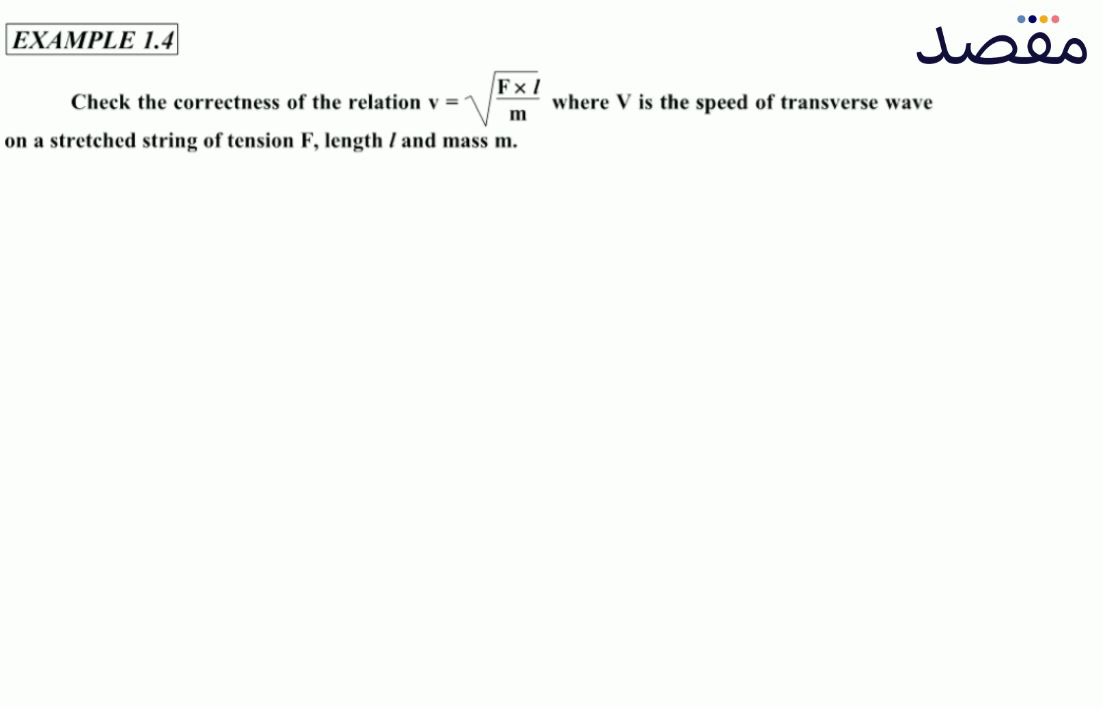# Classes

Change the way you learn with Maqsad's classes. Local examples, engaging animations, and instant video solutions keep you on your toes and make learning fun like never before!

Class 9Class 10First YearSecond Year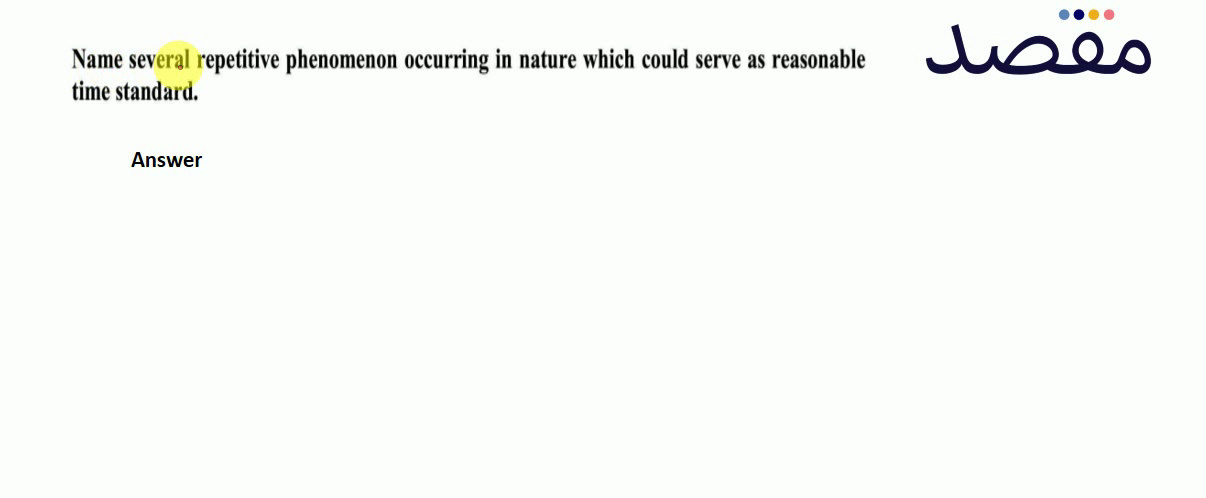### Name several repetitive phenomenon occurring in nature which could serve as reasonable time standard.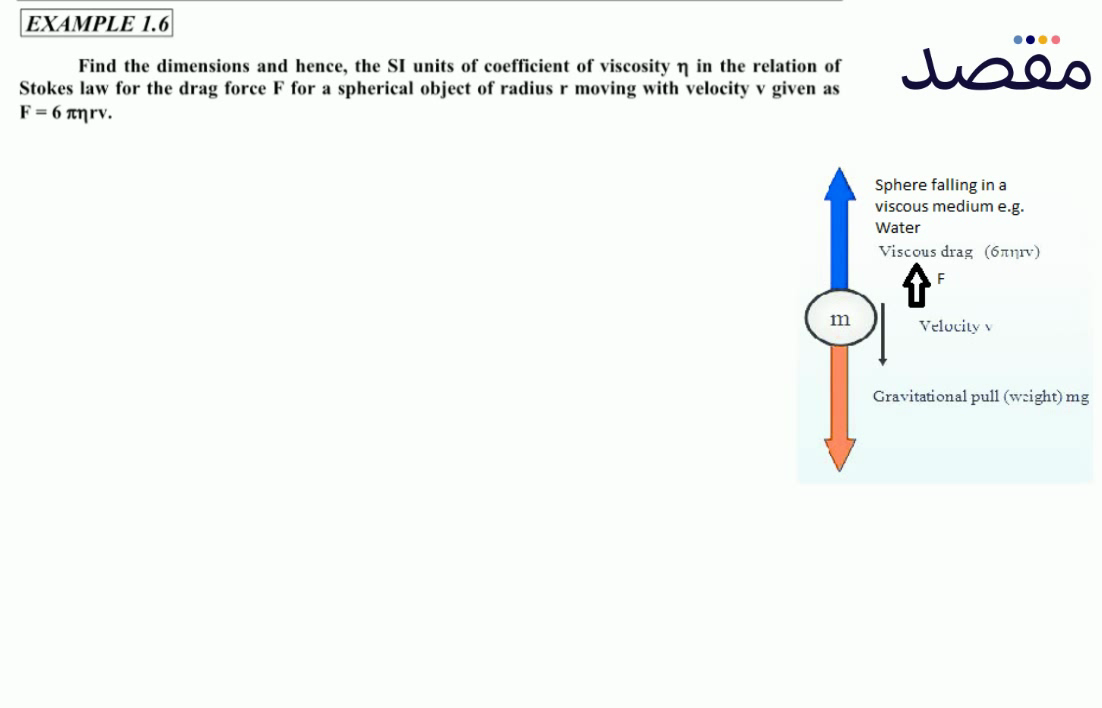### EXAMPLE 1.6Find the dimensions and hence the SI units of coefficient of viscosity \eta in the relation of Stokes law for the drag force F for a spherical object of radius r moving with velocity v given as F=6 \pi \eta rv.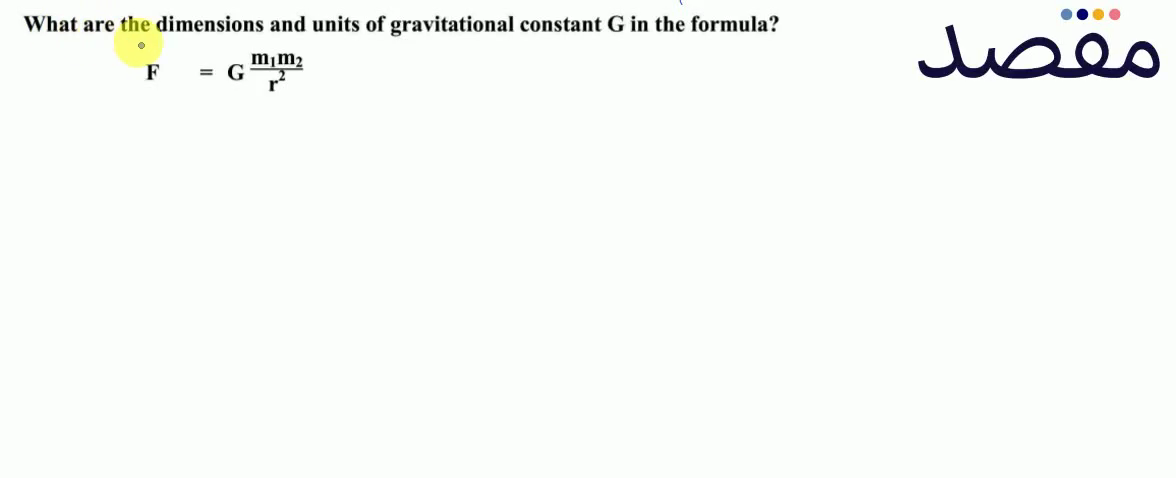### What are the dimensions and units of gravitational constant G in the formula?$F=G \frac{\mathbf{m}_{1} \mathbf{m}_{2}}{\mathbf{r}^{2}}$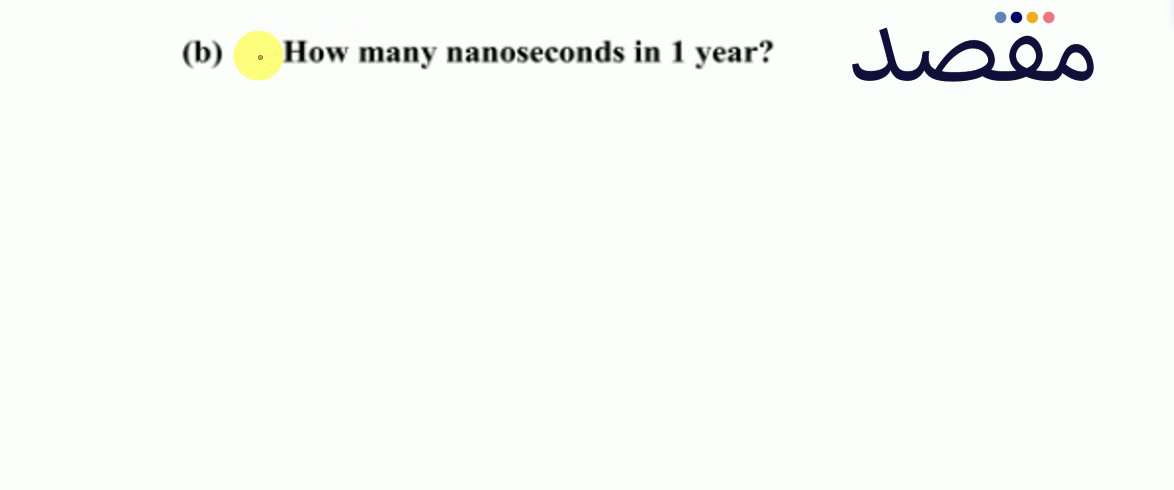### (b) How many nanoseconds in 1 year?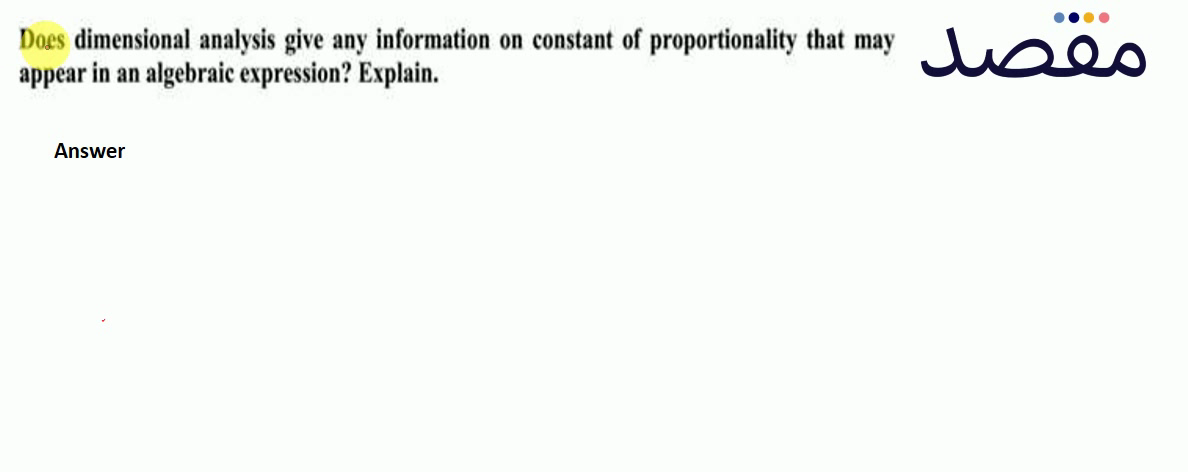### Does dimensional analysis give any information on constant of proportionality that may appear in an algebraic expression? Explain.